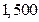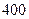Chapter 28, Problem 42P### Principles of Economics 2e

2nd Edition
Steven A. Greenlaw; David Shapiro
ISBN: 9781947172364

#### Solutions

Chapter
Section### Principles of Economics 2e

2nd Edition
Steven A. Greenlaw; David Shapiro
ISBN: 9781947172364
Textbook Problem

# If GDP isand the money supply iswhat is velocity?

To determine

The velocity of money, given the nominal GDP and money supply.

Explanation

The velocity of money is pace of circulation of money in the economy. The formula for calculating velocity of money is given below.

velocityofmoney=Nominal GDPMoneySupplyv=YMS

Given Information:

Money supply, MS= $400 Nominal GDP =$1500

### Still sussing out bartleby?

Check out a sample textbook solution.

See a sample solution

#### The Solution to Your Study Problems

Bartleby provides explanations to thousands of textbook problems written by our experts, many with advanced degrees!

Get Started

#### Find more solutions based on key concepts